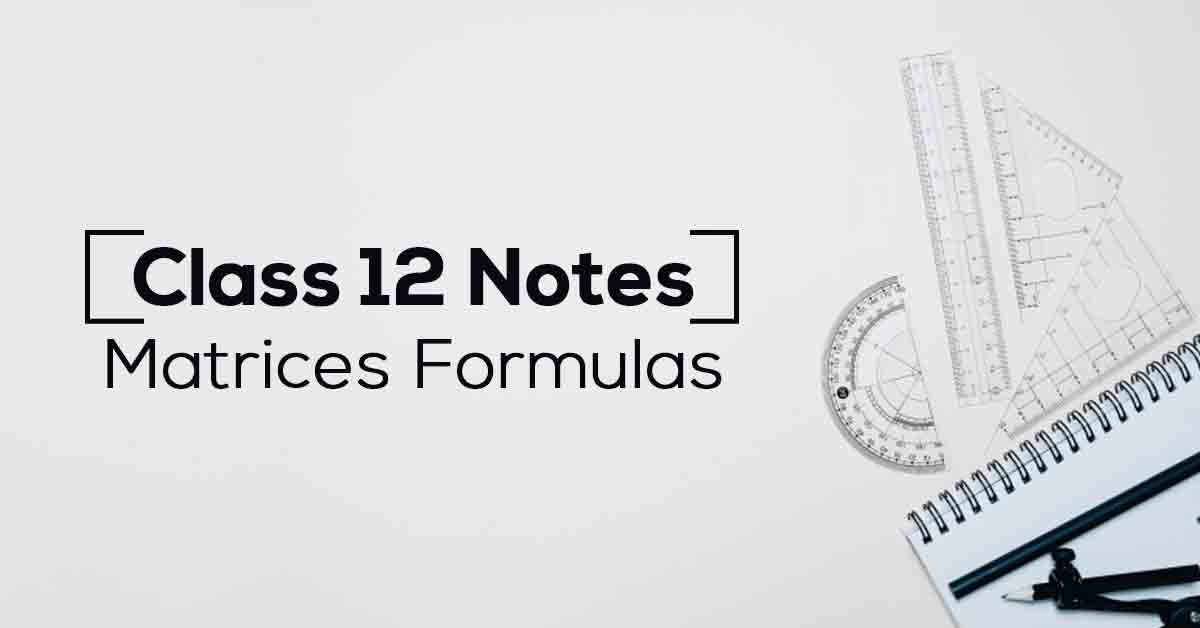Class 12th Math Matrices Formulas CBSE 2023

# Matrices Class 12 Formulas and Notes# Chapter 3 Matrices Formulas

Class 12 board exams are a turning point in a student’s life. Therefore, students must focus on practicing NCERT notes for Class 12 Maths chapters. In this article, students can find the Class 12 NCERT notes Maths Chapter 3 Matrices. It is critical for students to go through Matrices chapter 3 notes to answer all types of questions asked during board exams.

CBSE Class 12 Maths Chapter 3 talks about Types of Matrices, Equality of Matrices, Operations on Matrices, Addition and Multiplication of Matrices, Symmetric and Skew-Symmetric Matrices, and Invertible Matrices. To access free NCERT notes for Class 12 Chapter 3 Maths along with important topics, keep scrolling down!

### Points to Remember

Students can find the important points to remember for this chapter below.

• A set of numbers (real or imaginary) or symbols or expressions arranged in the form of a rectangular array of m rows and n columns is called an m×n matrix.

• A square matrix A=[aij]n×n is called an identity or a unit matrix, if aij=0 for all i≠j and aij=1 for all i=j.

• Commutativity: If A and B are two matrices of the same order, then A+B=B+A.

• Associativity: If A, B, and C are three matrices of the same order, then (A+B)+C=A+(B+C).

• Existence of Identity: The null matrix is the identity element for matrix addition i.e., A+O=A=O+A

• Existence of Inverse: For every matrix A=[aij]m×n, there exists a matrix −A=[−aij]m×n, such that A+(−A)=O=(−A)+A

• Cancellation Laws: If A, B, C are three matrices of the same order, then A+B=A+C⇒B=C and, B+A=C+A⇒B=C

• In order to find the inverse of a non-singular square matrix A by elementary operations, we write A=IA or A=AI

• We perform a sequence of elementary row operations successively on A on the LHS and the pre-factor I on RHS till we obtain. The matrix B, so obtained, is the desired inverse of matrix A.

### Topics and Sub-topics

Matrices is the most interesting chapters of all the topics provided in Class 12 Maths. Once the basics of the topic are understood, students will find problem-solving a fun-loving experience. Furthermore, practicing the solutions will become easy and smooth.

Vidyakul offers NCERT notes to every question provided at the end of the textbooks designed considering the latest syllabus. Moreover, students can practice the questions at Vidyakul for free to achieve higher marks in their exams.

Below, we have provided the list of topics included in this chapter:

 S.No Topic Name 1 Basic of Matrices 2 Types of Matrices 3 Operation on Matrices 4 Symmetric and Skew-Symmetric Matrices 5 Elementary Operation (Transformation of a Matrix) 6 Inverse Matrix

Download the FREE PDF of Matrices Class 12 Formulas and Notes and start your preparation with Vidyakul!